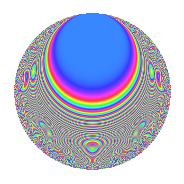# Properties

 Label 349.2.cLevel 349 Weight 2 Character orbit c Rep. character $$\chi_{349}(122,\cdot)$$ Character field $$\Q(\zeta_{3})$$ Dimension 56 Newforms 1 Sturm bound 58 Trace bound 0

# Related objects

## Defining parameters

 Level: $$N$$ = $$349$$ Weight: $$k$$ = $$2$$ Character orbit: $$[\chi]$$ = 349.c (of order $$3$$ and degree $$2$$) Character conductor: $$\operatorname{cond}(\chi)$$ = $$349$$ Character field: $$\Q(\zeta_{3})$$ Newforms: $$1$$ Sturm bound: $$58$$ Trace bound: $$0$$

## Dimensions

The following table gives the dimensions of various subspaces of $$M_{2}(349, [\chi])$$.

Total New Old
Modular forms 60 60 0
Cusp forms 56 56 0
Eisenstein series 4 4 0

## Trace form

 $$56q - 30q^{4} + 4q^{5} + 14q^{6} + 2q^{7} + 6q^{8} - 28q^{9} + O(q^{10})$$ $$56q - 30q^{4} + 4q^{5} + 14q^{6} + 2q^{7} + 6q^{8} - 28q^{9} - 2q^{10} - 2q^{11} + 11q^{12} - 2q^{13} + 2q^{14} + 9q^{15} - 34q^{16} + 18q^{18} - 5q^{19} + 14q^{20} + 12q^{21} - 7q^{22} - 11q^{23} - 30q^{24} - 6q^{25} - 11q^{26} - 30q^{27} - 52q^{28} + 8q^{29} - 21q^{30} - 48q^{31} - 6q^{32} + 12q^{33} - 14q^{34} + 42q^{35} + 66q^{36} + 14q^{37} + 60q^{38} - 26q^{39} + 24q^{40} - 3q^{42} - 23q^{43} - 20q^{44} + 18q^{45} + 5q^{46} - 26q^{47} - 22q^{48} - 26q^{49} + 11q^{50} + 14q^{51} + 6q^{52} - 12q^{53} - 7q^{54} + 10q^{55} - 19q^{56} + 25q^{57} - 12q^{58} - 16q^{59} - 12q^{60} + 42q^{61} - 27q^{62} + 31q^{63} + 54q^{64} + 72q^{65} - 66q^{66} - 34q^{67} - 57q^{68} + 10q^{69} - 52q^{70} - 10q^{71} + 47q^{72} + 23q^{73} - 17q^{74} - 26q^{75} + 9q^{76} - 10q^{77} + 25q^{78} + 48q^{79} - 32q^{80} - 12q^{81} - 8q^{82} + 14q^{83} + 10q^{84} - 3q^{85} + 46q^{86} + 14q^{87} + 58q^{88} + 8q^{89} + 68q^{90} + 54q^{91} + 48q^{92} - 57q^{93} + 33q^{94} + 54q^{95} - 72q^{96} + 32q^{98} + 73q^{99} + O(q^{100})$$

## Decomposition of $$S_{2}^{\mathrm{new}}(349, [\chi])$$ into irreducible Hecke orbits

Label Dim. $$A$$ Field CM Traces $q$-expansion
$$a_2$$ $$a_3$$ $$a_5$$ $$a_7$$
349.2.c.a $$56$$ $$2.787$$ None $$0$$ $$0$$ $$4$$ $$2$$